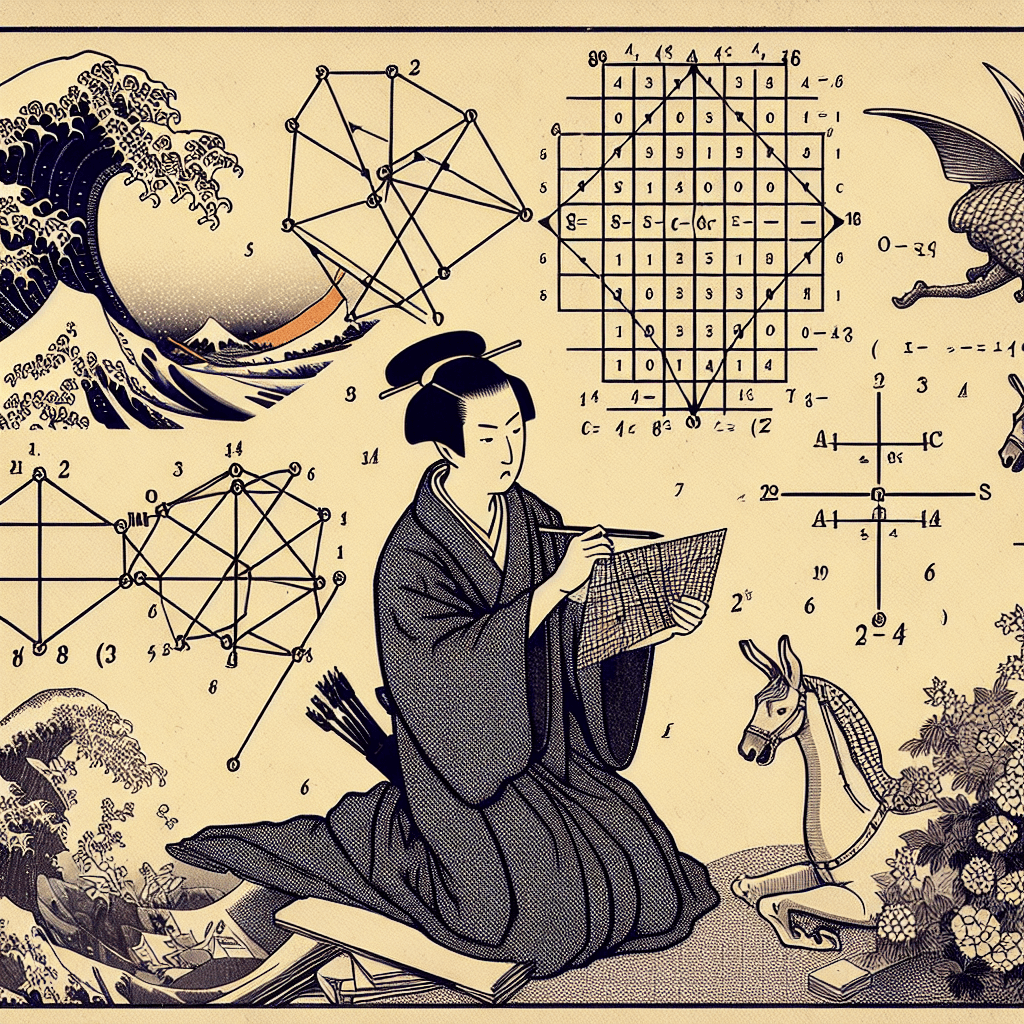# Linear Algebra in Neural Networks: A Comprehensive Introduction

syndu | June 6, 2023, 2:41 p.m.# Linear Algebra in Neural Networks: A Comprehensive Introduction

## Introduction

Linear algebra is a fundamental branch of mathematics that plays a crucial role in the functioning of neural networks. In this blog post, we'll explore the basics of linear algebra, including vectors, matrices, and common operations, and discuss how these concepts are relevant to neural networks. We'll also touch upon the role of matrix inversion in neural networks.

## Vectors and Matrices

Vectors are one-dimensional arrays of numbers that can represent quantities with both magnitude and direction. Matrices, on the other hand, are two-dimensional arrays of numbers arranged in rows and columns. They can be thought of as collections of vectors.

## Linear Algebra Operations

1. Addition and subtraction: To add or subtract two matrices or vectors, they must have the same dimensions. You add or subtract corresponding elements.
2. Scalar multiplication: To multiply a matrix or vector by a scalar (a single number), you multiply each element by the scalar.
3. Matrix multiplication: To multiply two matrices, the number of columns in the first matrix must equal the number of rows in the second matrix. The result is a matrix with the same number of rows as the first matrix and the same number of columns as the second matrix.
4. Matrix inversion: The inverse of a square matrix is a matrix that, when multiplied by the original matrix, results in the identity matrix. Not all matrices have inverses; a matrix must be non-singular (i.e., its determinant is non-zero) to have an inverse.

## Matrix Inversion in Neural Networks

While matrix inversion is not a core operation in the forward and backward propagation steps of neural networks, it can be relevant in certain optimization methods and specific cases. For example, in linear regression and second-order optimization methods, matrix inversion or its approximation may be used. However, due to its computational expense, especially for large matrices, matrix inversion is not commonly used in deep learning.

## Conclusion

Understanding the basics of linear algebra is essential for anyone interested in neural networks and deep learning. As you delve deeper into the subject, you'll encounter more advanced concepts and operations, such as eigenvectors and eigenvalues. The fundamentals outlined in this blog post will serve as a solid foundation for your further studies.

"Linear algebra is a fundamental branch of mathematics that plays a crucial role in the functioning of neural networks."

##### Lilith's Grimoire

The app you are using right now is Lilith's Grimoire

The Grimoire is full of Source Code. It is alive, and running, and you can explore the way it is built

The first lesson uncovers all of it.#### Understanding Matrix Determinants, Cofactor Expansion, and Systems of Linear Equations#### Mastering Matrices: An Overview of Their Properties and Operations#### Mastering the Basics: An Introduction to Vectors#### Vector Spaces: The Foundation of High-Dimensional Data in Language Models#### Key Linear Algebra Concepts for Large Language Models#### Linear Algebra Concepts in Large Language Models#### Crucial Linear Algebra Topics in Large Language Models#### Basics of Linear Algebra: Vectors, Matrices, and Operations